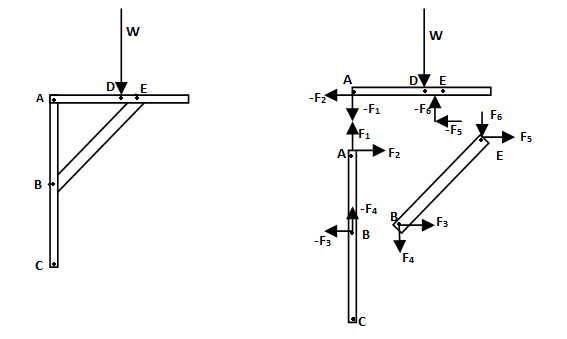The Basics of Mechanics

General Physics, High School and College Freshman levelIntroduction to Vectors and MotionVectors and Projectile MotionNewton's Laws of MotionWork, Force and EnergySimple Harmonic MotionRotational DynamicsFluid Mechanics

Mechanics comes to life in the world of Engineering: From Structures to Bridges to BuildingsCentroid And Center of Gravity - A Tutorial with Solved Problems - Centroids of Areas and Lines, Composite Areas, First Moments Analysis of Structures - Trusses, Method of Joints and Sections - A Tutorial with Solved Problems

General Physics, High School and College Freshman levelElectrostatics - Part 1: Introducing Columb's Law, Electric Field and Potential Gauss Theorem and Capacitance: Theory, definitions and problemsElectrostatics - Part 2: Some Interesting Problems and their Solutions Electromagnetism - Part 1: The Basics Lorentz Force, Bio-Savart law, Ampere's force law, basic laws related to Magnetic fields. Magnetic field intensities due to common current distributions. Electromagnetic Induction. Self and mutual induction. Electromagnetism - Part 2: Some interesting problems and their Solutions Advanced concepts in Electrostatics and Electromagnetism ( Theory only )Advanced concepts related to electrostatics and electromagnetism (theory only).

General Physics, High School and College Freshman levelCircuit Theory 1a- Introduction to Electrical Engineering, DC Circuits, Resistance and Capacitance, Kirchoff LawCircuit Theory 1b - More solved problems related to DC Circuits Star and Delta network transformations, maximum power transfer theorem, etc. Circuit Theory 2a - Introducing InductorsCircuit Theory 2b - Problems related to RL, LC, RLC circuitsCircuit Theory 3a - Electrical Networks and Network TheoremsCircuit Theory 3b - More network theorems, solved problems

General Physics, High School and College Freshman levelGeneral Physics, High School and College Freshman levelAn Introduction to Sound

Subpages (22):